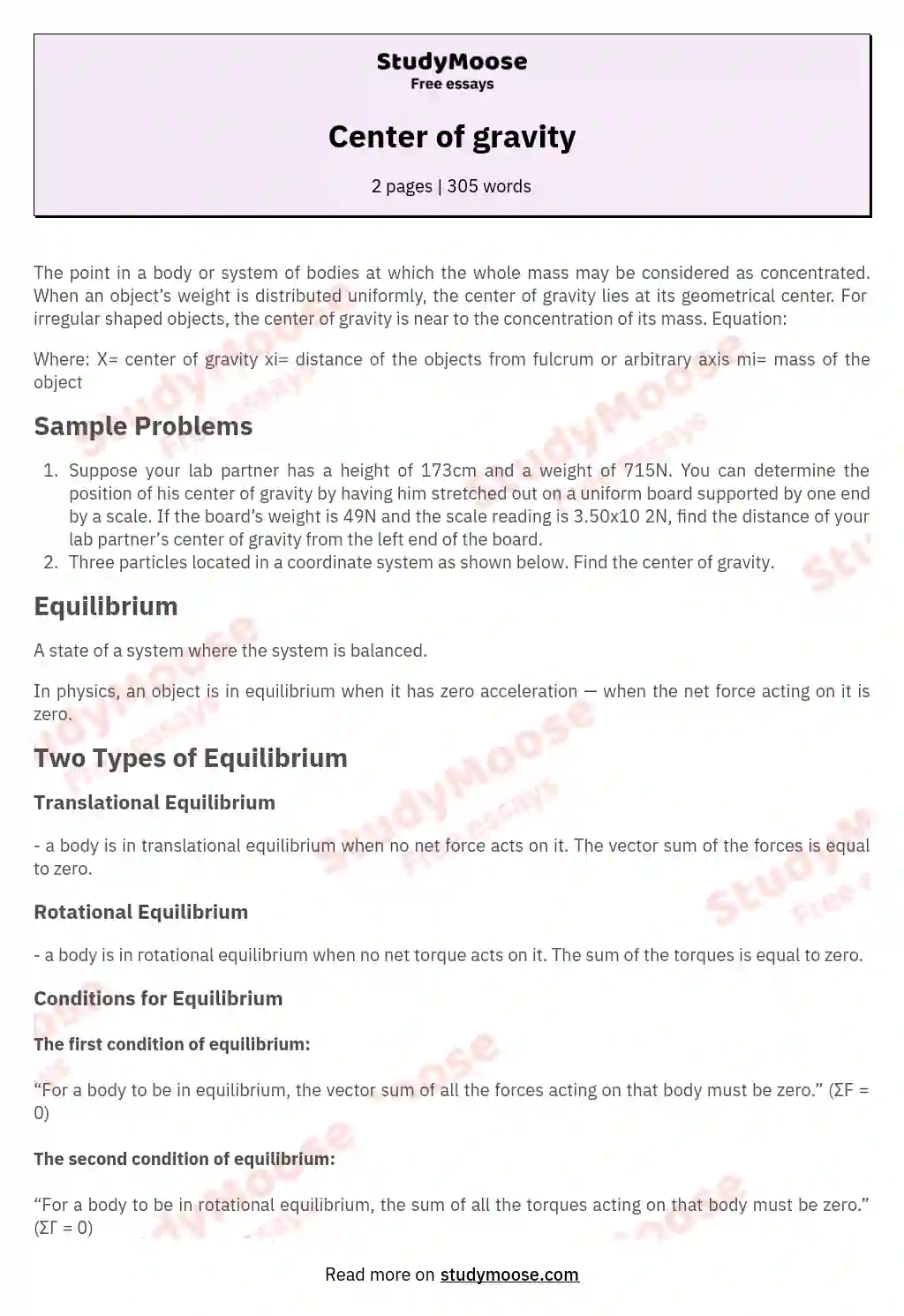# Center of gravity

Categories: Gravity

The point in a body or system of bodies at which the whole mass may be considered as concentrated. When an object’s weight is distributed uniformly, the center of gravity lies at its geometrical center. For irregular shaped objects, the center of gravity is near to the concentration of its mass. Equation:

Where:
X= center of gravity
xi= distance of the objects from fulcrum or arbitrary axis mi= mass of the object

## Sample Problems

1. Suppose your lab partner has a height of 173cm and a weight of 715N.

You can determine the position of his center of gravity by having him stretched out on a uniform board supported by one end by a scale. If the board’s weight is 49N and the scale reading is 3.50×10 2N, find the distance of your lab partner’s center of gravity from the left end of the board.

2. Three particles located in a coordinate system as shown below. Find the center of gravity.

Get quality help nowWriter LylaVerified writer

Proficient in: Gravity5 (876)

“ Have been using her for a while and please believe when I tell you, she never fail. Thanks Writer Lyla you are indeed awesome ”+84 relevant experts are online

## Equilibrium

A state of a system where the system is balanced.

In physics, an object is in equilibrium when it has zero acceleration — when the net force acting on it is zero.

## Two Types of Equilibrium

### Translational Equilibrium

– a body is in translational equilibrium when no net force acts on it. The vector sum of the forces is equal to zero.

### Rotational Equilibrium

– a body is in rotational equilibrium when no net torque acts on it. The sum of the torques is equal to zero.

### Conditions for Equilibrium

#### The first condition of equilibrium:

“For a body to be in equilibrium, the vector sum of all the forces acting on that body must be zero.

Get to Know The Price Estimate For Your Paper
Topic
Number of pages
Email Invalid email

You won’t be charged yet!

” (ΣF = 0)

#### The second condition of equilibrium:

“For a body to be in rotational equilibrium, the sum of all the torques acting on that body must be zero.” (ΣΓ = 0)